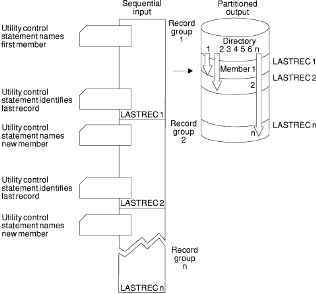## Saturday, 10 August 2013

### JCL IEBGENER Utility | IEBGENER Utility Examples.IBM IEBGENER Utility.

Today's tutorial is all about a popular mainframe utility. I would cover various aspect and daily use of this utility. Can you guess the name of that Utility ?

You guess is correct, it's IEBGENER utility, it is popular mainframe utility. The IEBGENER utility provides an easy mechanism to copy a non-VSAM sequential data set.

## What is IEBGENER Utility?

In layman term, IEBGENER utility is a sequential copy / generate data set program. You can use IEBGENER to perform below mention daily tasks:

• IEBGENER utility in mainframe create a backup of sequential data set (files) or PDSE.
• IEBGENER utility can be use to print non-VSAM sequential data-set by copying it to SYSOUT.
• IEBGENER utility can be use to rearrange fields and convert their data formats.
• IEBGENER utility can amplify an existing partitioned data set (PDS).
• IEBGENER utility can change or re-block the data set record logical length. Copy user labels on sequential output data sets.IEBGENER Utility Creating PDS from sequential data set.

Below mention figure, explain the process of creating partitioned data set from a sequential data-set. The sequential data-set is represent by left section of figure.

IEBGENER utility statement are use split sequential data-set into logical records groups. Finally, the right section of fig represent partitioned data-set.

### Let's discuss couple of IEBGENER Utility in Mainframe Examples.

#### IEBGENER Utility Example : 1 Blocked output.

//RXTC02T JOB ... (JOB CARD)
//STEP1    EXEC PGM=IEBGENER
//SYSPRINT DD SYSOUT=*
//SYSIN    DD DUMMY
//SYSUT2   DD DSNAME=OUTSET,UNIT=tape,LABEL=(,SL),
//            DISP=(,KEEP),VOLUME=SER=091234,
//            DCB=(RECFM=FB,LRECL=80,BLKSIZE=2000)
//SYSUT1   DD *
(in-stream data)
/*

The job control statements are as follows:
• SYSIN DD defines a dummy data set.
• SYSUT2 DD defines the output data set, OUTSET.
• SYSUT1 DD defines the in-stream data which is actually a JES SYSIN data set. The data set contains no statements.

#### Example : 2 IEBGENER Utility to print a sequential data set.

//RTX012R JOB ... (JOB CARD)
//STEP01   EXEC PGM=IEBGENER
//SYSPRINT DD SYSOUT=*
//SYSIN    DD DUMMY
//SYSUT1   DD DSNAME=RCD80.DATA,DISP=SHR
//SYSUT2   DD SYSOUT=A

#### Example : 3 A job step that uses IEBGENER to copy a sequential file.

//RC01RX    JOB ...... (JOB CARD)
//STEP01    EXEC  PGM=IEBGENER
//SYSPRINT  DD    SYSOUT=*
//SYSUT1    DD    DSNAME=RC01.CUSTMAST,DISP=SHR
//SYSUT2    DD    DSNAME=RC01.CUSTMAST.BKP,DISP=(NEW,CATLG),
//                UNIT=SYSDA,SPACE=(CYL,(10,10))
//SYSIN     DD    DUMMY

#### Example : 4 A job step that uses IEBGENER to print a sequential file

//RC01RX    JOB ...... (JOB CARD)
//STEP01    EXEC  PGM=IEBGENER
//SYSPRINT  DD    SYSOUT=*
//SYSUT1    DD    DSNAME=RC01.PAYROL,DISP=SHR
//SYSUT2    DD    SYSOUT=*
//SYSIN     DD    DUMMY

#### Example : 5 A job step that uses IEBGENER to copy a sequential file with reformatting

//RCTX012  JOB....... (JOB CARD)
//STEP01    EXEC  PGM=IEBGENER
//SYSPRINT  DD    SYSOUT=*
//SYSUT1    DD    DSNAME=RC01.PAYMAST,DISP=SHR
//SYSUT2    DD    DSNAME=RC01.PAYMAST.TEST,DISP=(NEW,CATLG),
//                UNIT=SYSDA,SPACE=(CYL,(1,1)),
//                DCB=(RECFM=FB,LRECL=40,BLKSIZE=800)
//SYSIN     DD    *
GENERATE MAXFLDS=5,MAXLITS=4
RECORD FIELD=(5,1,,1),FIELD=(20,21,,6),FIELD=(9,61,ZP,26),  X
FIELD=(9,70,ZP,31),FIELD=(4,'TEST',,36)
/*

Created with Artisteer